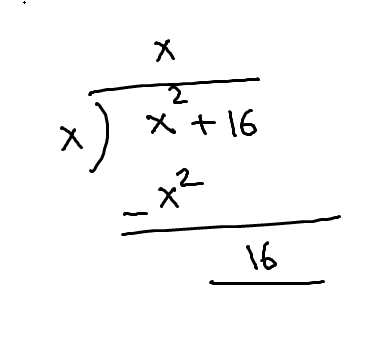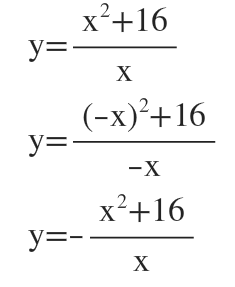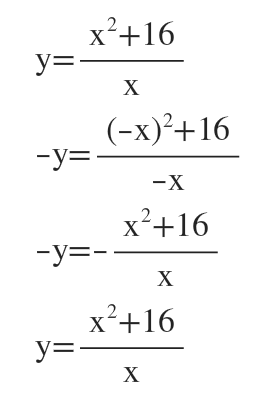# 7.f(x)= x^2+16/xA) select the correct choice a) the equation of the slant asymptote is ___b) there is no slant aymptoteB) Determine the symmetry of the grapha) neither y-axis symmetry nor origin symmetryb)origin symmetry c)y-axis symmetry C) What is the y-intercept?a) the y-intercept is ___b) there is no y-interceptPlot points x-6-5567f(x)= x^2+16/x      Graph:

Question
1 views

7.

f(x)= x^2+16/x

A) select the correct choice

• a) the equation of the slant asymptote is ___
• b) there is no slant aymptote

B) Determine the symmetry of the graph

• a) neither y-axis symmetry nor origin symmetry
• b)origin symmetry
• c)y-axis symmetry

C) What is the y-intercept?

• a) the y-intercept is ___
• b) there is no y-intercept

Plot points

 x -6 -5 5 6 7 f(x)= x^2+16/x

Graph:

check_circle

Step 1

Since we only answer up to 3 sub-parts, we’ll answer the first 3. Please resubmit the question and specify the other subparts (up to 3) you’d like answered.

a) The degree of the numerator is 2 and the degree of the denominator is 1.

Since the degree of the numerator is 1 more than the degree of the denominator, so there will be a slant asymptote.

to find slant asymptote we use the long division.The quotient is x, so y=x is the slant asymptote.

Answer(a): a) the equation of the slant asymptote is y=x

Step 2

b) To check y axis symmetry we have to plug -x in place of x.The equation has changed so there is no y-axis symmetry.

To check origin symmetry we have to plug -x in place of x and -y in place of y.The equation did not change, so it has symmetry about the origin

...

### Want to see the full answer?

See Solution

#### Want to see this answer and more?

Solutions are written by subject experts who are available 24/7. Questions are typically answered within 1 hour.*

See Solution
*Response times may vary by subject and question.
Tagged in